## Why are scripts useful?

They facilitate reproducible science: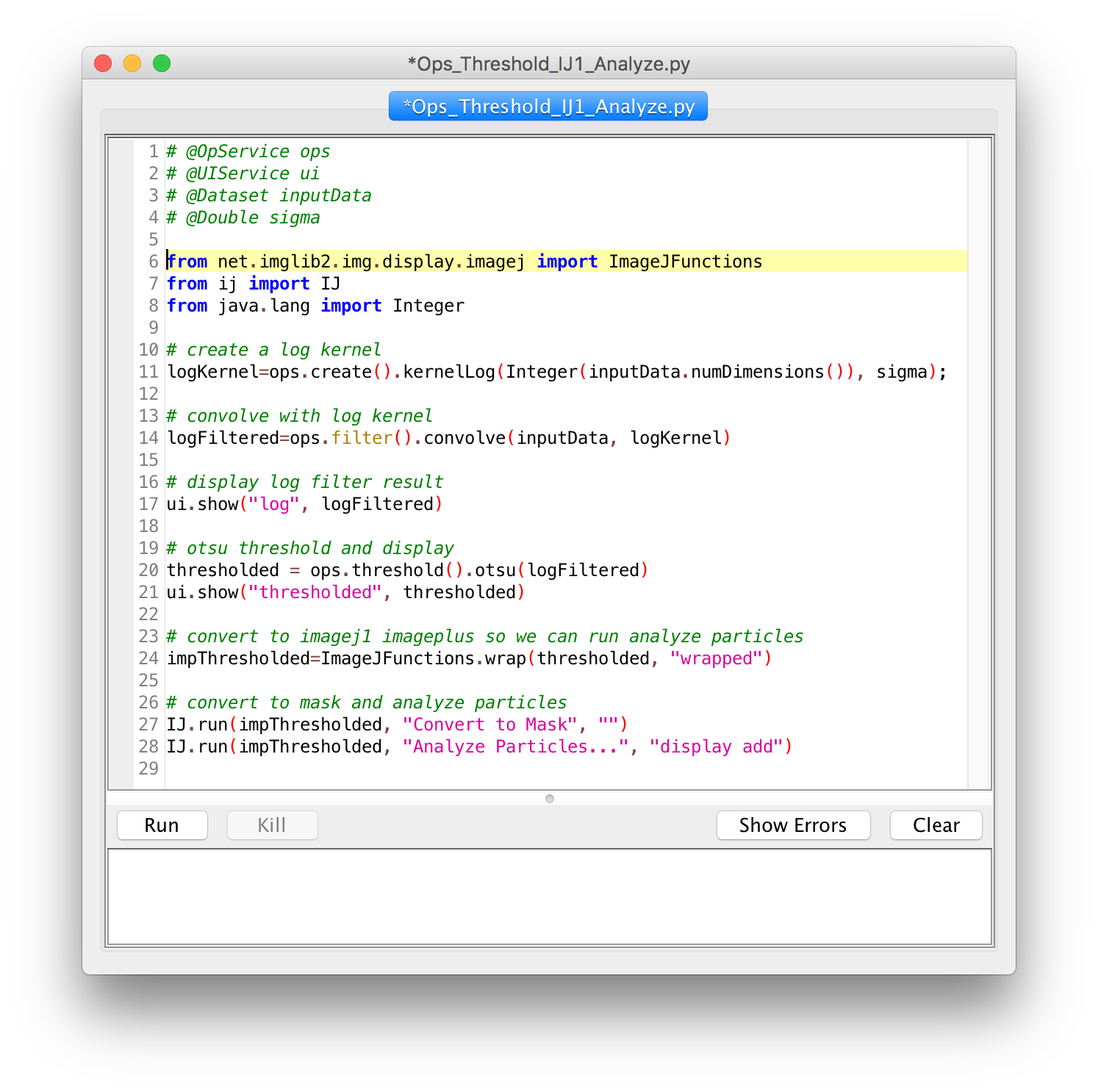• Document your work
• Automate your analysis
• Share with the world

## Script Editor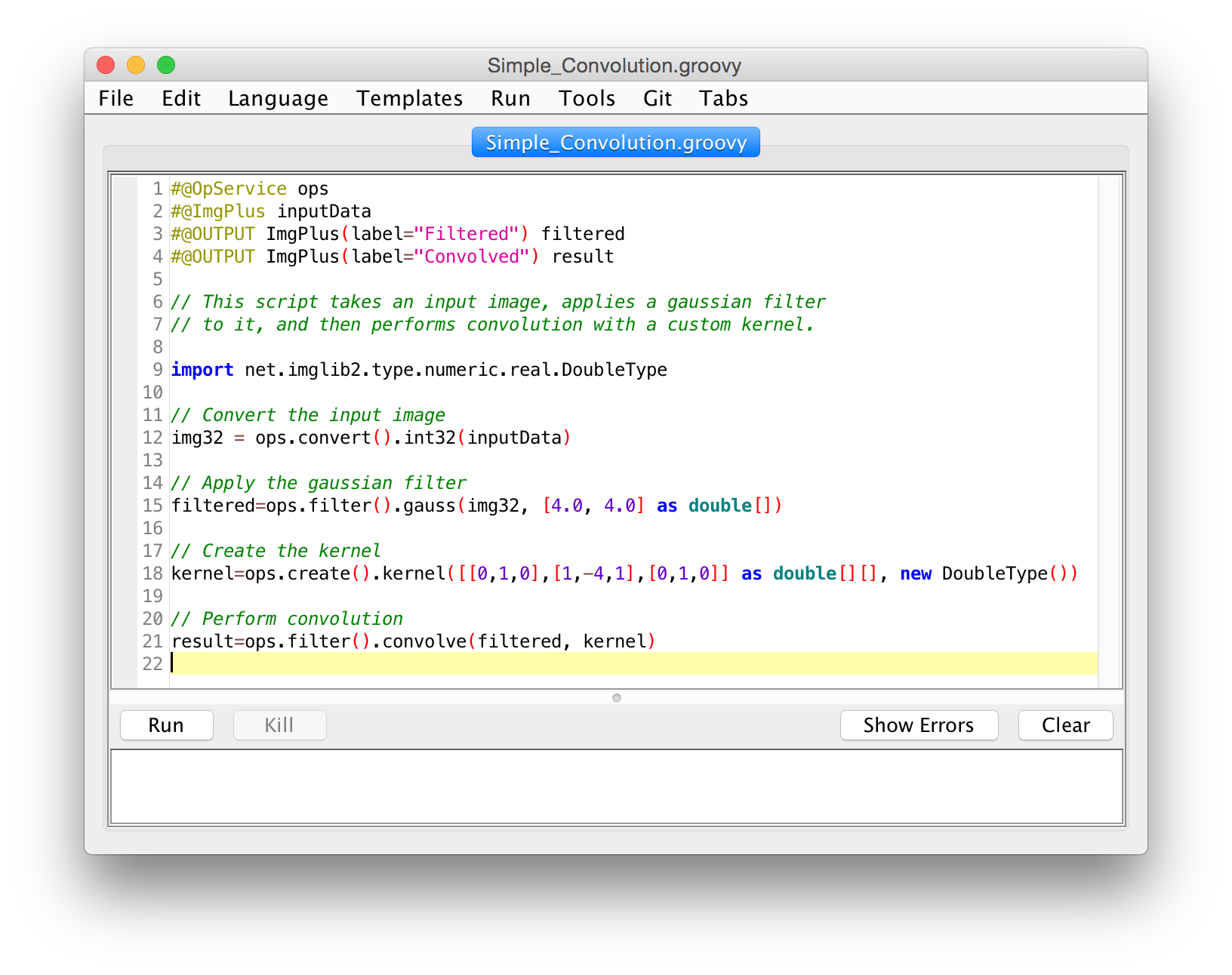• Comments
• Variables
• Functions
• String manipulation
• Conditionals
• Loops

## Macros

### Comments

``````
// Comments allow you to put human-readable thoughts
// into your code.

// The goal of this "macro" is simply to teach you about comments!

// Comments help you to remember why you did something:
// Set the value to "2" because my boss said so!
value = 2; // Comments can be added to any line!

// Code can be disabled by commenting it out:
// x = y * 2;
``````

### Variables

What is a variable?

• Open "Blobs" image (File > Open Samples > Blobs (25K))
• ``````
run("Blue");
run("Fire");
``````

### Variables

What is a variable?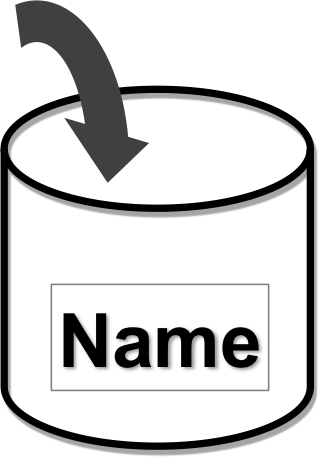• Container
• "A piece of code that can vary"
• ``````
color = "Blue";
run(color);
``````
• Two Types: Numbers or Strings

### Variables

``````
// what sorts of values can we assign?
title = "Hello, World!";                // string
intensity = 255;                        // number
a = exp(x * sin(y)) + atan(x * y – a);  // expression

// string constant vs. variable name
text = "title";
text = title;

x = 3;
y = x;
x = 5; // what is the value of y after this?

// the variable is assigned after the expression is evaluated
intensity = intensity * 2;
``````

### Strings

``````
name = "copy";

// you can concatenate strings, and strings and numbers
text = "The name is " + name;

// numbers versus strings and more concatenation...
a = 2;
b = 3;
print(a + b);

a = "2";
b = "3";
print(a + b);

// what happens when we run this?
run("Duplicate...", "title=name");

// what's different with this line?
run("Duplicate...", "title=" + name);
``````

### Functions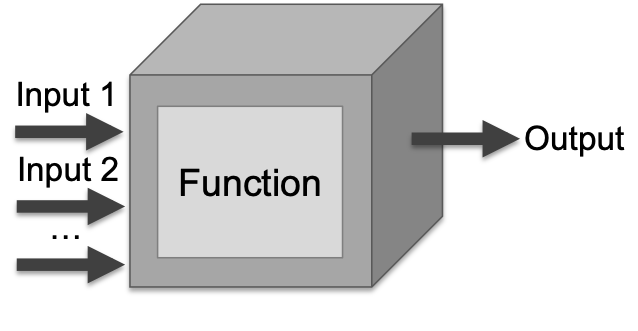``````

function myFunction(arguments) {
// Your code goes here
}

``````
``````

function setLUT(lutName) {
run(lutName);
}

``````

### Functions

``````
print("Hello, world!");

// functions can return values
// Hint: use parameters instead of calling getNumber
number = getNumber("Type in a number!", 5);

// the "run" function is the most important one
// it follows the structure of run("Command...", "Argument(s) String");
run("Duplicate...", "title=New");

// for arguments with spaces, enclose in square brackets
run("Duplicate...", "title=[with spaces]");
``````

### Conditionals

``````
#@String instructor

if (getBoolean("Is " + instructor + " going too fast?")) {
hint = "Tell them to to slow down!";
}
else {
hint = "Try to modify the code, play with it...";
}
showMessage("Advice:", hint);
``````

### Loops

``````
// For loops:
// They use assignment, test, increment
// Use when you know the # of times the loop will run
for (i = 1; i <= 10; i++) {
print("Counter: " + i);
}

// While loops:
// Use when you do not necessarily know the # of times the loop will run
while (getBoolean("Do you want me to keep going?")) {
print("Ok, I'm still going...");
}
showMessage("Ok, I'm done!");
``````

### Tying it all together

Do you notice a bug in this code?

``````
// this example makes a stack of blurred versions of the
// current slice with a range of radii.

radius = getNumber("Maximal radius?", 5);

title = "Blurred stack of " + getTitle();
run("Duplicate...", "title=[" + title + "]");
run("Select All");
run("Copy");
for (i = 1; i <= radius; i++) {
run("Add Slice");
run("Paste");
run("Gaussian Blur...", "radius=" + radius);
}
``````

## Macro Recorder

1. Launch the Recorder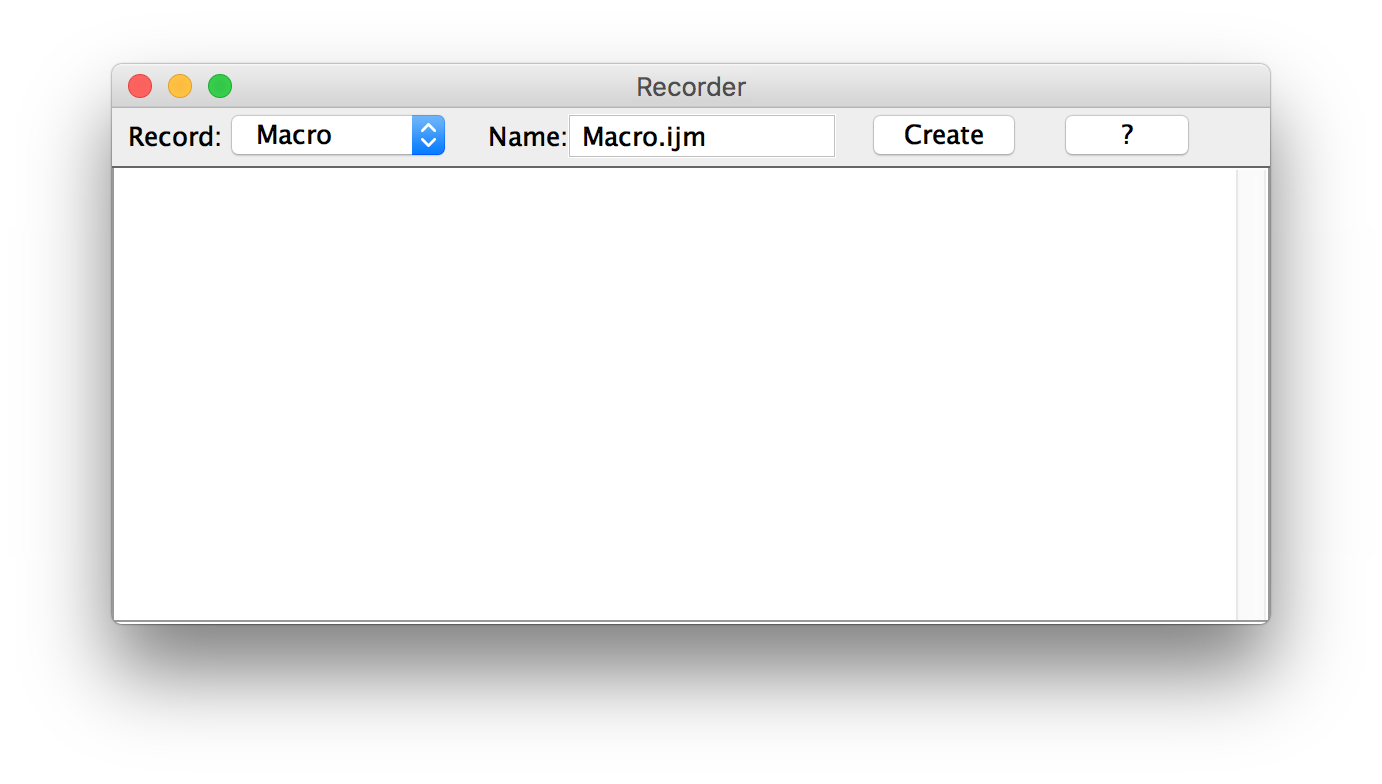• Use the Search Bar! (Ctrl+L)
• OR: click the Dev icon, then Record...
• OR: Plugins ▶ Macros ▶ Record...

2. Execute operations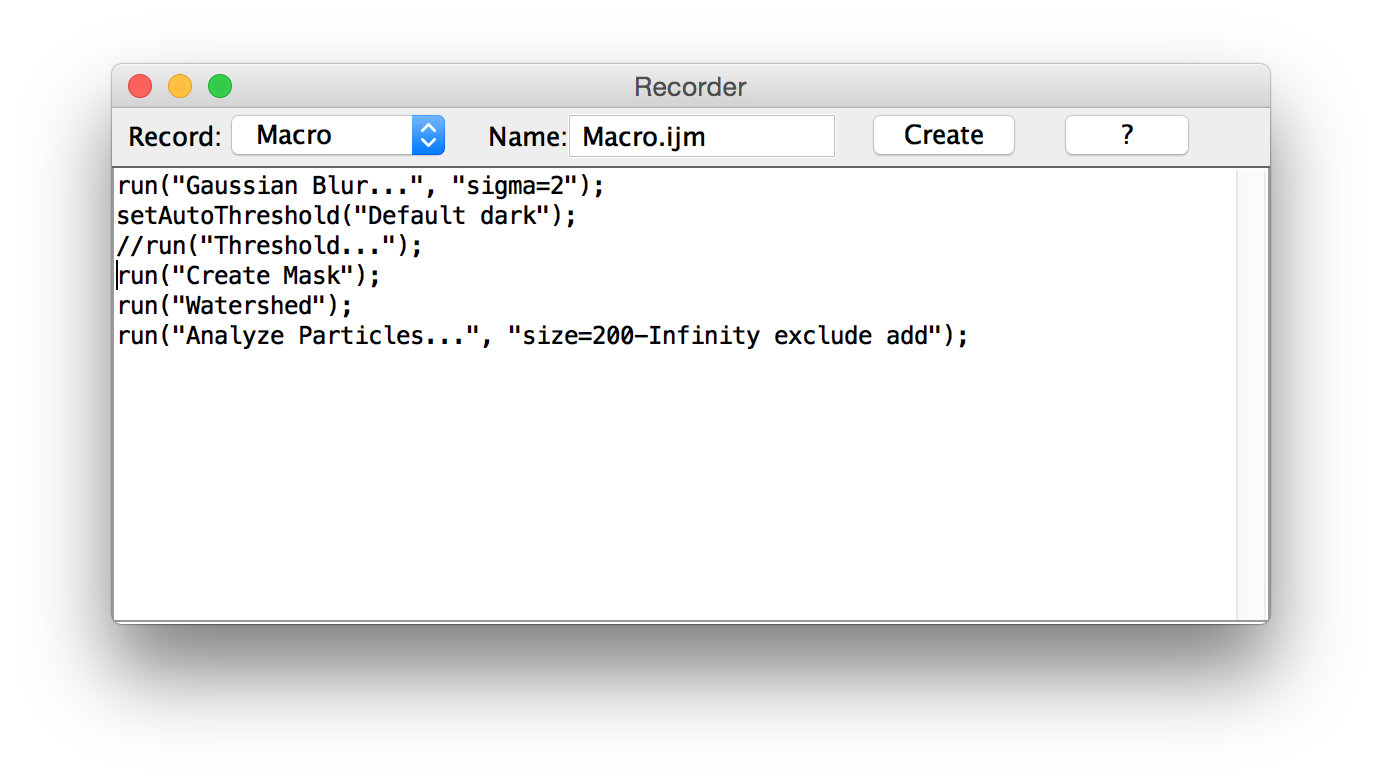Use the Command Finder (Ctrl+L)!

• Open sample images
• Download/unpack "images/C3-jw-30min.zip"
• Plugins ▶ Bio-Formats ▶ Bio-Formats Importer
• Open "C3-jw-30min 5_c5.tif"
• Apply Gaussian blur
• Apply a threshold (Shift+T)
• Create Mask
• Watershed
• Analyze Particles...
• Size: 200-Infinity
• Exclude on edges; Add to Manager

### Code Check!

``````
run("Gaussian Blur...", "sigma=2");
setAutoThreshold("Default dark");
//run("Threshold...");
run("Create Mask");
run("Watershed");
run("Analyze Particles...", "size=200-Infinity exclude add");
``````

3. Spruce it up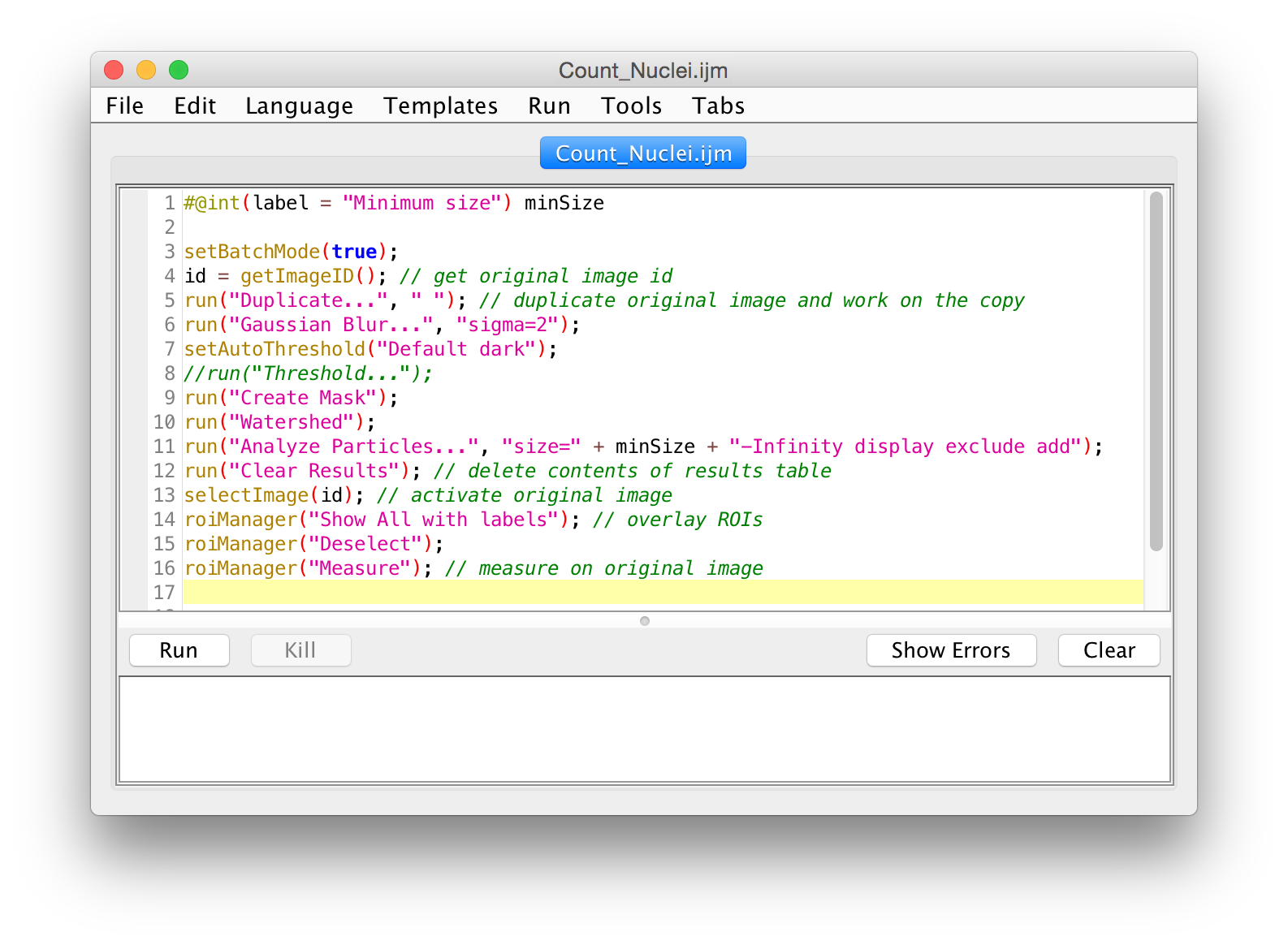• Click the "Create" button
• Use script parameters
• `setBatchMode(true)`
• Use image IDs
• Store in `scripts`
• In a folder matching the desired menu
• Use underscore for spaces

### Code Check!

``````
#@int(label = "Minimum size") minSize

setBatchMode(true);
id = getImageID(); // get original image id
run("Duplicate...", " "); // duplicate original image and work on the copy
run("Gaussian Blur...", "sigma=2");
setAutoThreshold("Default dark");
//run("Threshold...");
run("Create Mask");
run("Watershed");
run("Analyze Particles...", "size=" + minSize + "-Infinity display exclude add");
run("Clear Results"); // delete contents of results table
selectImage(id); // activate original image
roiManager("Show All with labels"); // overlay ROIs
roiManager("Deselect");
roiManager("Measure"); // measure on original image
``````

## Script parameters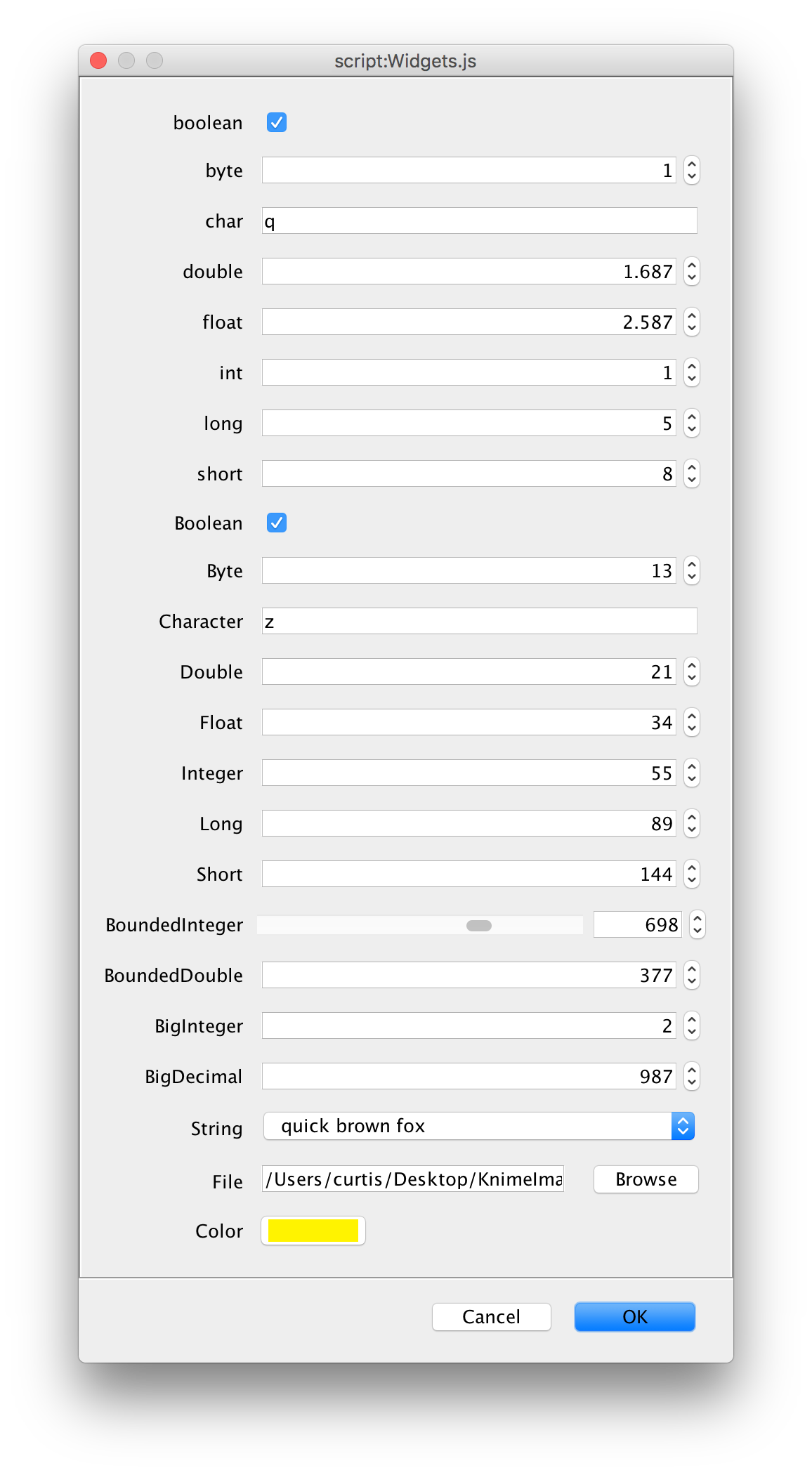• Templates ▶ Intro ▶ Widgets (BeanShell)
• Run
• Fix the bugs!
• To the `boundedInteger` parameter, add:
`style="scroll bar"`
• To the `string` parameter, add:
`choices={"quick fox", "lazy dog"}`
• Run again

## Batch processing

Process ▶ Batch ▶ Macro...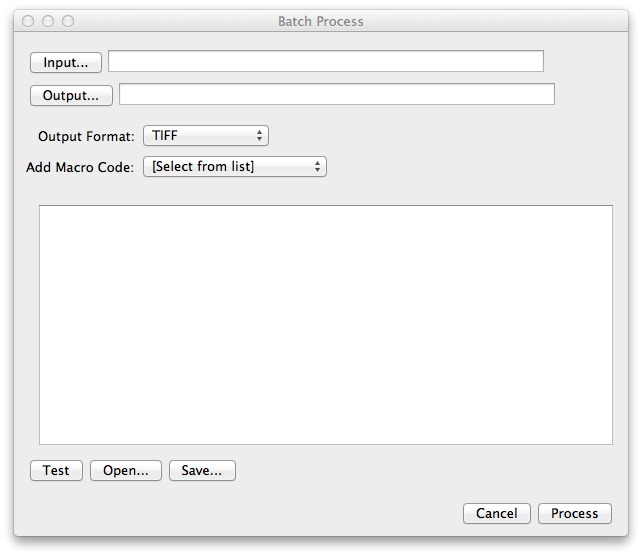Templates ▶ ImageJ 1.x ▶ Examples ▶ Process Folder (IJ1 Macro)Example script to count nuclei in multiple images in a folder/subfolders:

``````
/*
* Macro to count nuclei in multiple images in a folder/subfolders.
*/

#@File(label = "Input directory", style = "directory") input
#@File(label = "Output directory", style = "directory") output
#@String(label = "File suffix", value = ".tif") suffix
#@int(label = "Minimum size") minSize

processFolder(input);

// function to scan folders/subfolders/files to find files with correct suffix
function processFolder(input) {
list = getFileList(input);
for (i = 0; i < list.length; i++) {
if(File.isDirectory(input + File.separator + list[i]))
processFolder("" + input + File.separator + list[i]);
if(endsWith(list[i], suffix))
processFile(input, output, list[i]);
}
//saves results for all images in a single file
saveAs("Results", output + "/All_Results.csv");
}

function processFile(input, output, file) {
setBatchMode(true); // prevents image windows from opening while the script is running
// open image using Bio-Formats
run("Bio-Formats", "open=[" + input + "/" + file +"] autoscale color_mode=Default rois_import=[ROI manager] view=Hyperstack stack_order=XYCZT");
id = getImageID(); // get original image id
run("Duplicate...", " "); // duplicate original image and work on the copy

// create binary image
run("Gaussian Blur...", "sigma=2");
setAutoThreshold("Default dark");
//run("Threshold...");
run("Create Mask");
run("Watershed");
// save current binary image
save(output + "/Binary_OUTPUT_" + file);

run("Analyze Particles...", "size=" + minSize + "-Infinity exclude add");
selectImage(id); // activate original image
roiManager("Show All with labels"); // overlay ROIs
roiManager("Deselect");
roiManager("Measure"); // measure on original image

// save ROIs for current image
roiManager("Deselect");
roiManager("Save", output+ "/" + file + "_ROI.zip"); // saves Rois zip file
roiManager("Deselect");
roiManager("Delete"); // clear ROI Manager for next image
}
``````

## Top tips...

when writing ImageJ Scripts## Further reading

Help from the community is here:

Scripting guide:

Additional workshops and presentations: